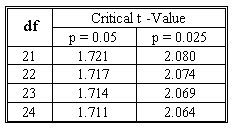### CFA Practice Question

There are 364 practice questions for this topic.

### CFA Practice Question

You have obtained the following sample regression equation from 25 samples:

y-hatt = 2.304 + 3.412Xt1 - 4.657X2t

The estimated standard errors are 0.87, 1.23 and 1.78 for b0, b1 and b2, respectively. Let α = 0.05. Would you reject H0: β1 <= 0 against H1: β1 > 0?The degrees of freedom: 25 - 2 - 1 = 22.
t-statistic = (3.412 - 0)/1.23 = 2.77.
The critical value of t having 22 degrees of freedom is 1.717.
As 2.77 > 1.717, we would reject the null hypothesis.

User Comment
soniamoren But the t value is calculated with the standard error not the standard deviation
katybo that is right!
danlan2 use p=0.05 since it's one tail test.
DBBrian If H0:B1=0, why it is not a two tail test? In addition, does it make sense that the null hypothese (B1=0) and the alternative hypothese (B1 > 0) not covering all scenario. How about (B1 < 0) ?
Vonoko 2.77 is way out - no need to think about the two-tailed or not. Besides n is 25. Generally, if n>30 t-statistic critical values are close to z-critical values (standard normal). For z as you remember 1.96 is the 2.5%
cfaajay It is a Two tailed test and t he confidence interval at 5% level of significance will be +/- 2.074 ,and hence you will reject the null Hypothesis .whenever the null hypothesis include equality then test will be two tailed .

Vonoko ,you are right in saying that @ 5% two tailed z value will be 1.96 ,but as it is t test the confidence interval should bw wider and hence its +/- 2.074.

Stick to what you know.
Oksanata it's a one tail test, but the t-critical should be 2.074 (according to the logic of previous quest.1)